# pandas系列（一)Pandas基础

2020/04/21 15:46

### 文章目录

• 第1章 Pandas基础

• 查看Pandas版本

• 一、文件读取与写入

• 1. 读取

• （a）csv格式

• （b）txt格式

• （c）xls或xlsx格式

• 2. 写入

• （a）csv格式

• （b）xls或xlsx格式

• 二、基本数据结构

• 1. Series

• （a）创建一个Series

• 对于一个Series，其中最常用的属性为值（values），索引（index），名字（name），类型（dtype）

• （b）访问Series属性

• （c）取出某一个元素

• 将在第2章详细讨论索引的应用，这里先大致了解

• （d）调用方法

• Series有相当多的方法可以调用：

• 2. DataFrame

• （a）创建一个DataFrame

• （b）从DataFrame取出一列为Series

• （c）修改行或列名

• （d）调用属性和方法

• （e）索引对齐特性

• 这是Pandas中非常强大的特性，不理解这一特性有时就会造成一些麻烦

• （f）列的删除与添加

• 对于删除而言，可以使用drop函数或del或pop

• pop方法直接在原来的DataFrame上操作，且返回被删除的列，与python中的pop函数类似

• 可以直接增加新的列，也可以使用assign方法

• 但assign方法不会对原DataFrame做修改

• （g）根据类型选择列

• （h）将Series转换为DataFrame

• 使用T符号可以转置

• 三、常用基本函数

• 从下面开始，包括后面所有章节，我们都会用到这份虚拟的数据集

• 可以指定n参数显示多少行

• 2. unique和nunique

• unique显示所有的唯一值

• 3. count和value_counts

• count返回非缺失值元素个数

• value_counts返回每个元素有多少个

• 4. describe和info

• info函数返回有哪些列、有多少非缺失值、每列的类型

• describe默认统计数值型数据的各个统计量

• 可以自行选择分位数

• 对于非数值型也可以用describe函数

• 5. idxmax和nlargest

• idxmax函数返回最大值，在某些情况下特别适用，idxmin功能类似

• nlargest函数返回前几个大的元素值，nsmallest功能类似

• 6. clip和replace

• clip和replace是两类替换函数

• clip是对超过或者低于某些值的数进行截断

• replace是对某些值进行替换

• 通过字典，可以直接在表中修改

• 7. apply函数

• apply是一个自由度很高的函数，在第3章我们还要提到

• 对于Series，它可以迭代每一列的值操作：

• 对于DataFrame，它可以迭代每一个列操作：

• 四、排序

• 1. 索引排序

• 2. 值排序

• 多个值排序，即先对第一层排，在第一层相同的情况下对第二层排序

# 第1章 Pandas基础

import pandas as pdimport numpy as np

#### 查看Pandas版本

pd.__version__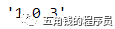## 一、文件读取与写入

### 1. 读取

#### （a）csv格式

df = pd.read_csv('data/table.csv')df.head()#### （b）txt格式

df_txt = pd.read_table('data/table.txt')   #可设置sep分隔符参数df_txt.head()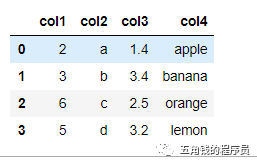#### （c）xls或xlsx格式

#需要安装xlrd包df_excel = pd.read_excel('data/table.xlsx')df_excel.head()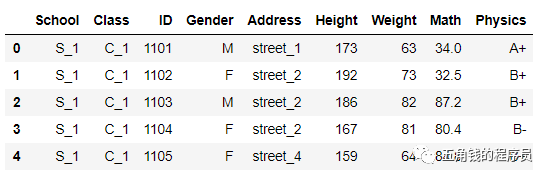### 2. 写入

#### （a）csv格式

df.to_csv('data/new_table.csv')#df.to_csv('data/new_table.csv', index=False) #保存时除去行索引

#### （b）xls或xlsx格式

#需要安装openpyxldf.to_excel('data/new_table2.xlsx', sheet_name='Sheet1')

## 二、基本数据结构

### 1. Series

#### 对于一个Series，其中最常用的属性为值（values），索引（index），名字（name），类型（dtype）

s = pd.Series(np.random.randn(5),index=['a','b','c','d','e'],name = '这是一个Series',dtype='float64')s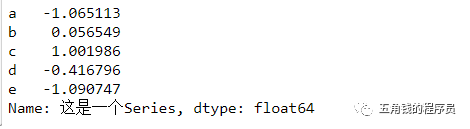#### （b）访问Series属性

s.values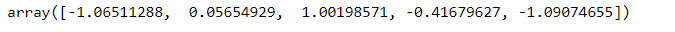s.name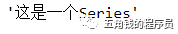s.index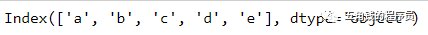#### 将在第2章详细讨论索引的应用，这里先大致了解

s['a']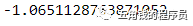#### （d）调用方法

s.mean()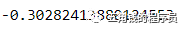#### Series有相当多的方法可以调用：

print([attr for attr in dir(s) if not attr.startswith('-')])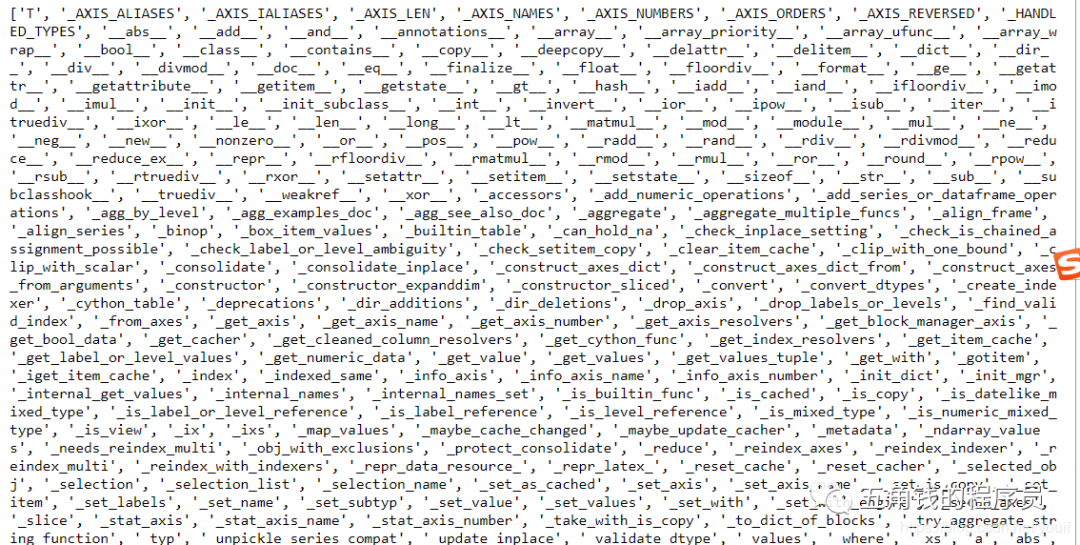### 2. DataFrame

#### （a）创建一个DataFrame

df = pd.DataFrame({'col1':list('abcde'),'col2':range(5,10),'col3':[1.3,2.5,3.6,4.6,5.8]},                 index = list('一二三四五'))df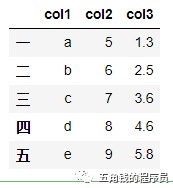#### （b）从DataFrame取出一列为Series

df['col1']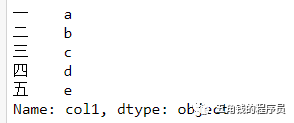type(df)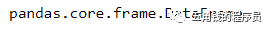type(df['col3'])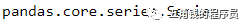#### （c）修改行或列名

df.rename(index={'一':'one'},columns={'col1':'new_col1'})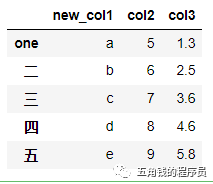#### （d）调用属性和方法

df.index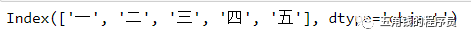df.columns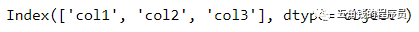df.values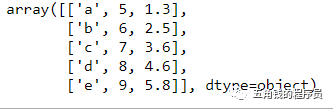df.shape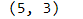df.mean()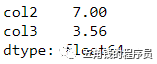#### 这是Pandas中非常强大的特性，不理解这一特性有时就会造成一些麻烦

df1 = pd.DataFrame({'A':[1,2,3]},index=[1,2,3])df2 = pd.DataFrame({'A':[1,2,3]},index=[3,1,2])df1 - df2#### 对于删除而言，可以使用drop函数或del或pop

df.drop(index='五',columns='col1')#设置inplace=True后会直接在原DataFrame中改动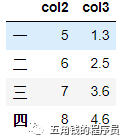df['col1']=[1,2,3,4,5]del df['col1']df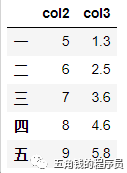#### pop方法直接在原来的DataFrame上操作，且返回被删除的列，与python中的pop函数类似

df['col1']=[1,2,3,4,5]df.pop('col1')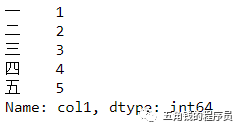df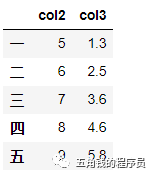#### 可以直接增加新的列，也可以使用assign方法

df1['B']=list('abc')df1.assign(C=pd.Series(list('def')))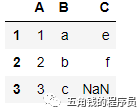#### 但assign方法不会对原DataFrame做修改

df1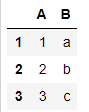#### （g）根据类型选择列

df.select_dtypes(include=['number']).head()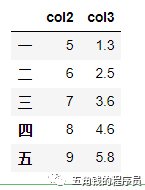df.select_dtypes(include=['float']).head()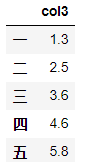#### （h）将Series转换为DataFrame

s = df.mean()s.name='to_DataFrame's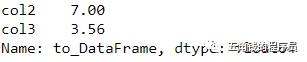s.to_frame()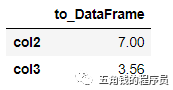#### 使用T符号可以转置

s.to_frame().T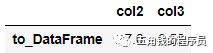## 三、常用基本函数

#### 从下面开始，包括后面所有章节，我们都会用到这份虚拟的数据集

df = pd.read_csv('data/table.csv')

df.head()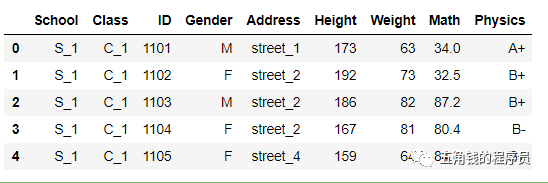df.tail()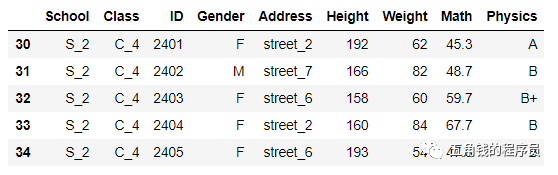#### 可以指定n参数显示多少行

df.head(3)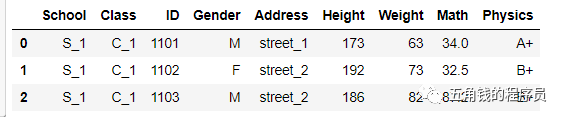### 2. unique和nunique

df['Physics'].nunique()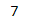#### unique显示所有的唯一值

df['Physics'].unique()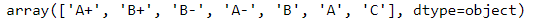### 3. count和value_counts

#### count返回非缺失值元素个数

df['Physics'].count()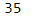#### value_counts返回每个元素有多少个

df['Physics'].value_counts()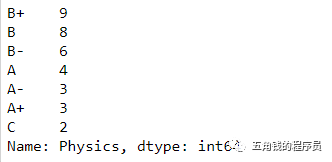### 4. describe和info

#### info函数返回有哪些列、有多少非缺失值、每列的类型

df.info()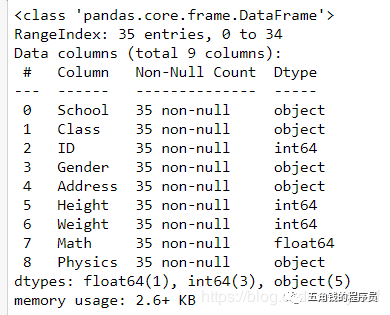#### describe默认统计数值型数据的各个统计量

df.describe()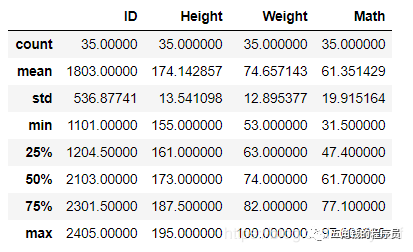#### 可以自行选择分位数

df.describe(percentiles=[.05, .25, .75, .95])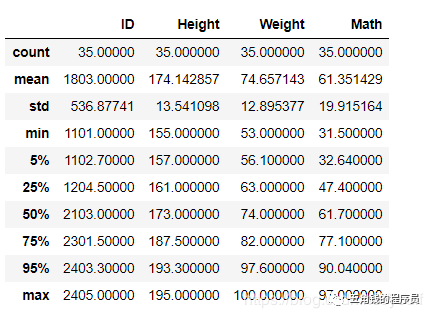#### 对于非数值型也可以用describe函数

df['Physics'].describe()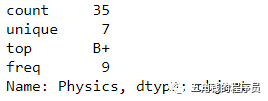### 5. idxmax和nlargest

#### idxmax函数返回最大值，在某些情况下特别适用，idxmin功能类似

df['Math'].idxmax()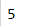#### nlargest函数返回前几个大的元素值，nsmallest功能类似

df['Math'].nlargest(3)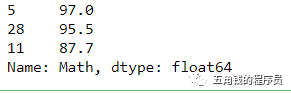### 6. clip和replace

#### clip是对超过或者低于某些值的数进行截断

df['Math'].head()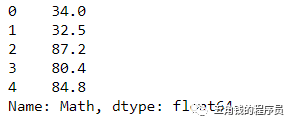df['Math'].clip(33,80).head()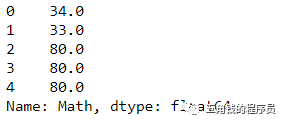df['Math'].mad()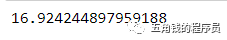#### replace是对某些值进行替换

df['Address'].head()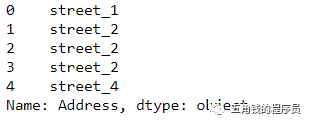df['Address'].replace(['street_1','street_2'],['one','two']).head()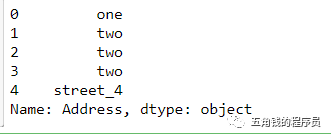#### 通过字典，可以直接在表中修改

df.replace({'Address':{'street_1':'one','street_2':'two'}}).head()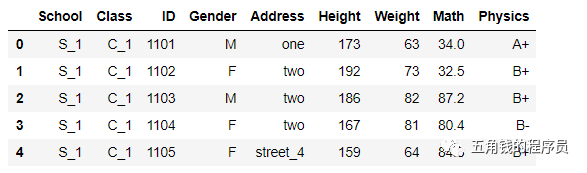### 7. apply函数

#### 对于Series，它可以迭代每一列的值操作：

df['Math'].apply(lambda x:str(x)+'!').head() #可以使用lambda表达式，也可以使用函数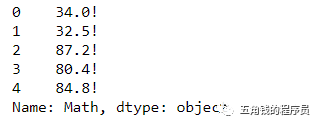#### 对于DataFrame，它可以迭代每一个列操作：

df.apply(lambda x:x.apply(lambda x:str(x)+'!')).head() #这是一个稍显复杂的例子，有利于理解apply的功能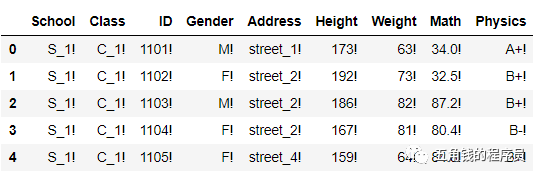## 四、排序

### 1. 索引排序

df.set_index('Math').head() #set_index函数可以设置索引，将在下一章详细介绍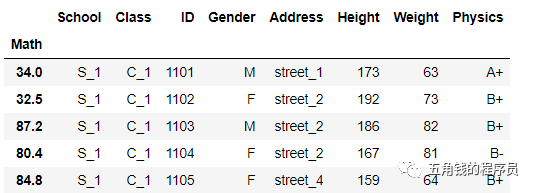df.set_index('Math').sort_index().head() #可以设置ascending参数，默认为升序，True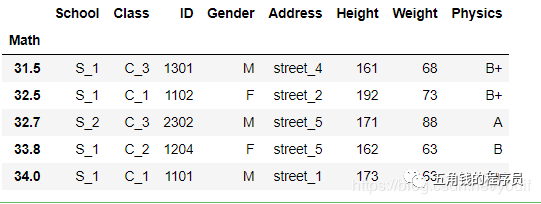### 2. 值排序

df.sort_values(by='Class').head()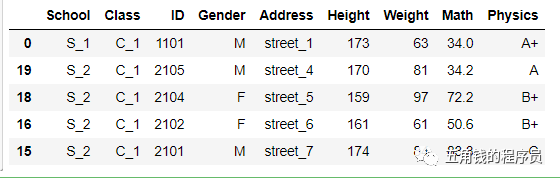#### 多个值排序，即先对第一层排，在第一层相同的情况下对第二层排序

df.sort_values(by=['Address','Height']).head()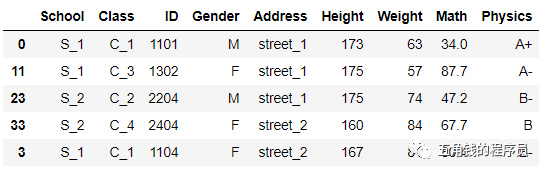https://github.com/XiangLinPro/pandas.git-Fear not that the life shall come to an end, but rather fear that it shall never have a beginning.——J.H. Newman
「不要害怕你的生活将要结束，应该担心你的生活永远不会真正开始。——纽曼」

0
0 收藏

### 作者的其它热门文章0 评论
0 收藏
0